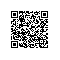# 好程序员web前端培训JavaScript学习笔记--jQuery

创建一个元素
var div = $(' ') 内部插入元素 // 向 div 元素中插入一个 p 元素，放在最后$('div').append($(' '))​// 把 p 元素插入到 div 中去，放在最后$('

hello

').appendTo($('div'))​// 向 div 元素中插入一个 p 元素，放在最前$('div').prepend($(' '))​// 把 p 元素插入到 div 中去，放在最前$('

hello

').prependTo($('div')) 外部插入元素 // 在 div 的后面插入一个元素 p$('div').after($(' '))​// 在 div 的前面插入一个元素 p$('div').before($(' '))​// 把 p 元素插入到 div 元素的后面$('div').insertAfter($(' '))​// 把 p 元素插入到 div 元素的前面$('div').insertBefore($(' ')) 替换元素 // 把 div 元素替换成 p 元素$('div').replaceWith($(' '))​// 用 p 元素替换掉 div 元素$('

').replaceAll($('div')) 删除元素 // 删除元素下的所有子节点$('div').empty()​// 把自己从页面中移除$('div').remove() 克隆元素 // 克隆一个 li 元素// 接受两个参数// 参数1： 自己身上的事件要不要复制，默认是 false// 参数2： 所有子节点身上的事件要不要复制，默认是 true$('li').clone()

操作元素的宽和高
// 获取 div 元素内容位置的高，不包含 padding 和 border$('div').height()// 设置 div 内容位置的高为 200px$('div').height(200)​// 获取 div 元素内容位置的宽，不包含 padding 和 border$('div').width()// 设置 div 内容位置的宽为 200px$('div').width(200)
获取元素的内置宽和高
// 获取 div 元素内容位置的高，包含 padding 不包含 border$('div').innerHeight()​// 获取 div 元素内容位置的宽，包含 padding 不包含 border$('div').innerWidth()
获取元素的外置宽和高
// 获取 div 元素内容位置的高，包含 padding 和 border$('div').outerHeight()// 获取 div 元素内容位置的高，包含 padding 和 border 和 margin$('div').outerHeight(true)​// 获取 div 元素内容位置的宽，包含 padding 和 border$('div').outerWidth()// 获取 div 元素内容位置的高，包含 padding 和 border 和 margin$('div').outerWidth(true)

元素相对页面的位置
// 获取 div 相对页面的位置$('div').offset() // 得到的是以一个对象 { left: 值, top: 值 }​// 给 div 设置相对页面的位置$('div').offset({ left: 100, top: 100 })// 获取定位到一个距离页面左上角 100 100 的位置
元素相对于父元素的偏移量
// 获取 div 相对于父元素的偏移量（定位的值）$('div').position() 获取页面卷去的高度和宽度 window.onscroll = function () { // 获取浏览器卷去的高度 console.log($(window).scrollTop())}​window.onscroll = function () {
// 获取浏览器卷去的宽度 console.log($(window).scrollLeft())} 元素事件 绑定事件的方法 // 给 button 按钮绑定一个点击事件$('button').on('click', function () {
console.log('我被点击了')})​// 给 button 按钮绑定一个点击事件，并且携带参数$('button').on('click', { name: 'Jack' }, function (e) { console.log(e) // 所有的内容都再事件对象里面 console.log(e.data) // { name: 'Jack' }})​// 事件委托的方式给 button 绑定点击事件$('div').on('click', 'button', function () {
console.log(this) // button 按钮})​// 事件委托的方式给 button 绑定点击事件并携带参数$('div').on('click', 'button', { name: 'Jack' }, function (e) { console.log(this) // button 按钮 console.log(e.data)}) 移除事件 // 给 button 按钮绑定一个 点击事件，执行 handler 函数$('button').on('click', handler)​// 移除事件使用 off$('button').off('click', handler) 只能执行一次的事件 // 这个事件绑定再 button 按钮身上// 当执行过一次以后就不会再执行了$('button').one('click', handler)
直接触发事件
// 当代码执行到这里的时候，会自动触发一下 button 的 click 事件$('button').trigger('click') 可以直接使用的常见事件 可以直接使用的事件就是可以不利用 on 来绑定，直接就可以使用的事件方法 click // 直接给 div 绑定一个点击事件$('div').click(function () {
console.log('我被点击了')})​// 给 div 绑定一个点击事件并传递参数$('div').click({ name: 'Jack' }, function (e) { console.log(e.data)}) dblclick // 直接给 div 绑定一个双击事件$('div').dblclick(function () {
console.log('我被点击了')})​// 给 div 绑定一个双击事件并传递参数$('div').dblclick({ name: 'Jack' }, function (e) { console.log(e.data)}) scroll // 直接给 div 绑定一个滚动事件$('div').scroll(function () {
console.log('我被点击了')})​// 给 div 绑定一个滚动事件并传递参数$('div').scroll({ name: 'Jack' }, function (e) { console.log(e.data)}) hover // 这个事件要包含两个事件处理函数// 一个是移入的时候，一个是移出的时候触发$('div').hover(function () {
console.log('我会再移入的时候触发')}, function () {
console.log('我会在移出的时候触发')})

show
// 给 div 绑定一个显示的动画$('div').show() // 如果元素本身是 display none 的状态可以显示出来​// 给 div 绑定一个显示的动画// 接受三个参数//$('div').show('毫秒', '速度', '回调函数') $('div').show(1000, 'linear', function () { console.log('我显示完毕')}) hide // 给 div 绑定一个隐藏的动画$('div').hide() // 如果元素本身是 display block 的状态可以隐藏起来​// 给 div 绑定一个显示的动画// 接受三个参数// $('div').show('毫秒', '速度', '回调函数')$('div').hide(1000, 'linear', function () {
console.log('我隐藏完毕')})
toggle
// 给 div 绑定一个切换的动画$('div').hide() // 元素本身是显示，那么就隐藏，本身是隐藏那么就显示​// 给 div 绑定一个显示的动画// 接受三个参数//$('div').show('毫秒', '速度', '回调函数') $('div').toggle(1000, 'linear', function () { console.log('动画执行完毕')}) animate // 定义一个自定义动画$('.show').click(function () {
$('div').animate({ width: 500, height: 300 }, 1000, 'linear', function () { console.log('动画运动完毕') })}) stop // 立刻定制动画$('div').stop() // 就停止再当前状态
finish
// 立刻结束动画\$('div').finish() // 停止在动画结束状态使用钉钉扫一扫加入圈子
+ 订阅